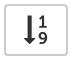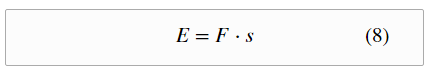# MathJax Equation Numbering¶

Enable automatic equation numbering and reset numbering upon pressing toolbar button.

A LaTeX equation like:

\begin{equation}
E = F \cdot s
\end{equation}


generates the numbered equation:## Internals¶

Equation numbering is activated this way:

MathJax.Hub.Config({
TeX: { equationNumbers: { autoNumber: "AMS" } }
});


Equation numbers are reset and math equations rerendered using this code:

MathJax.Hub.Queue(
["resetEquationNumbers", MathJax.InputJax.TeX],
["PreProcess", MathJax.Hub],
["Reprocess", MathJax.Hub]
);